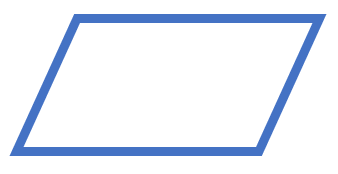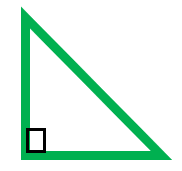# Math Worksheets Land

Math Worksheets For All Ages

# Math Worksheets Land

Math Worksheets For All Ages

# Constructing Geometric Shapes Worksheets

There are many different times in life when we will need to compose new figures to explain our surroundings. We do this when creating a map, explaining a play in sports, designing a new product, or building a new home. In fact, fabricators and architects basically do this for a living. There are two different types of figures 2 dimensional and 3 dimensional shapes. They also come in regular symmetrical forms and irregular forms where the sides and angles are asymmetrical. The goal of this section is for you to be able to do the first step of creating any blueprint. Students will use these worksheets and lessons to learn how to draw geometric shapes given a series of instructions.

### Aligned Standard: Grade 7 Geometry - 7.G.A.2

• Answer Keys - These are for all the unlocked materials above.

### Homework Sheets

For the homework we focus on triangles. They are only of those relevant shapes to this level.

• Homework 1 - Draw a quadrilateral with 4 sides, with opposite sides parallel with no right angles.
• Homework 2 - Will the lengths of all three sides of each example below create a triangle? Explain how you know which will work.
• Homework 3 - An isosceles triangle with only one 50° angle. Find the two angles.

### Practice Worksheets

The practice sheets don't stray too far from the homework.

• Practice 1 - Draw a two dimensional shape made by drawing a curve that is always the same distance from a center.
• Practice 2 - Will the lengths of all three sides of each example below create a triangle? Explain how you know which will work
• Practice 3 - Draw a quadrilateral which has non-parallel sides equal and its base angles are equal?

### Math Skill Quizzes

My favorite has always been the, "Do You Have A Triangle?" quiz.

• Quiz 1 - Draw an Octagon.
• Quiz 2 - Will the lengths of all three sides of each example below create a triangle?
• Quiz 3 - Draw a triangle with all sides of different length. No sides are equal and no angles are equal.

### How to Draw Geometric Shapes from Given Instructions

Drawing geometric shapes is not a very difficult thing to go. There is a set of pre-requisites that are required when forming the general foundation of any geometric shape. Different shapes require different instructions and data that need to be handled accordingly. A shape of any kind is a closed area within a boundary. They differ by a combination of curves, points, lines, sides, and angles.

For example, if you were asked to draw a parallelogram. Then you must have the basic information required for understanding the figure. First and foremost, you need to know that a parallelogram is a quadrilateral (meaning four-sided figure) with two opposite sides parallel and equal in length.

Similarly, if you are asked to draw a triangle with one ninety-degree angle, that implies that you have been asked to draw a right-angled triangle. Given this angle, we can use the Pythagoras to understand the remaining two angles of the triangle will be less than 90-degree.

You need to basic pieces of information to create a drawing of a shape based on given instructions:

a) The angle(s) of the figure.

b) The specific number of sides of a figure.

The tools that you need to put together these figures very accurately would include a compass (for anything with curves), a ruler, and a protractor (for your angles). I highly recommend jotting down what the instructions you are given tell are requirements. We put this into motion for you as an example below:

Problem - Draw a parallelogram and a right-triangle.

Solution - This problem is asking use to create two figures. We are given a good bit of freedom here on the size of the shapes because it is not specified for either figure. Here is what each shape requires:

1) Parallelogram – These are four sided figures where the opposite sides are parallel. A good example would be:2) Right-triangle - A triangle has three sides. A right-triangle has one angle that is at ninety degrees (right angle). So, we make sure to specific the right angle in the figure. This is often done by drawing a square at the point of the angle as you see in the diagram below:Unlock all the answers, worksheets, homework, tests and more!
Save Tons of Time! Make My Life Easier Now

## Thanks and Don't Forget To Tell Your Friends!

I would appreciate everyone letting me know if you find any errors. I'm getting a little older these days and my eyes are going. Please contact me, to let me know. I'll fix it ASAP.